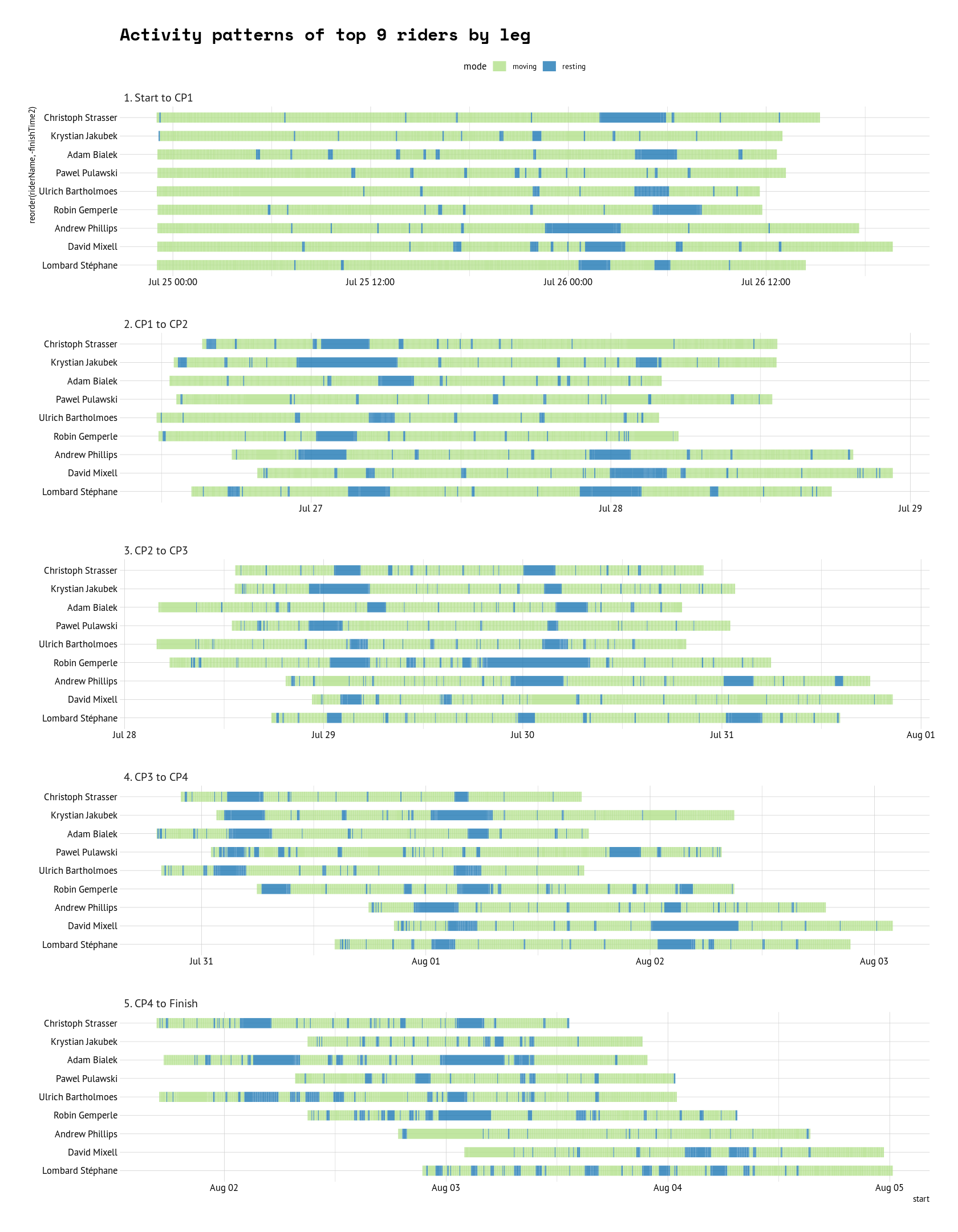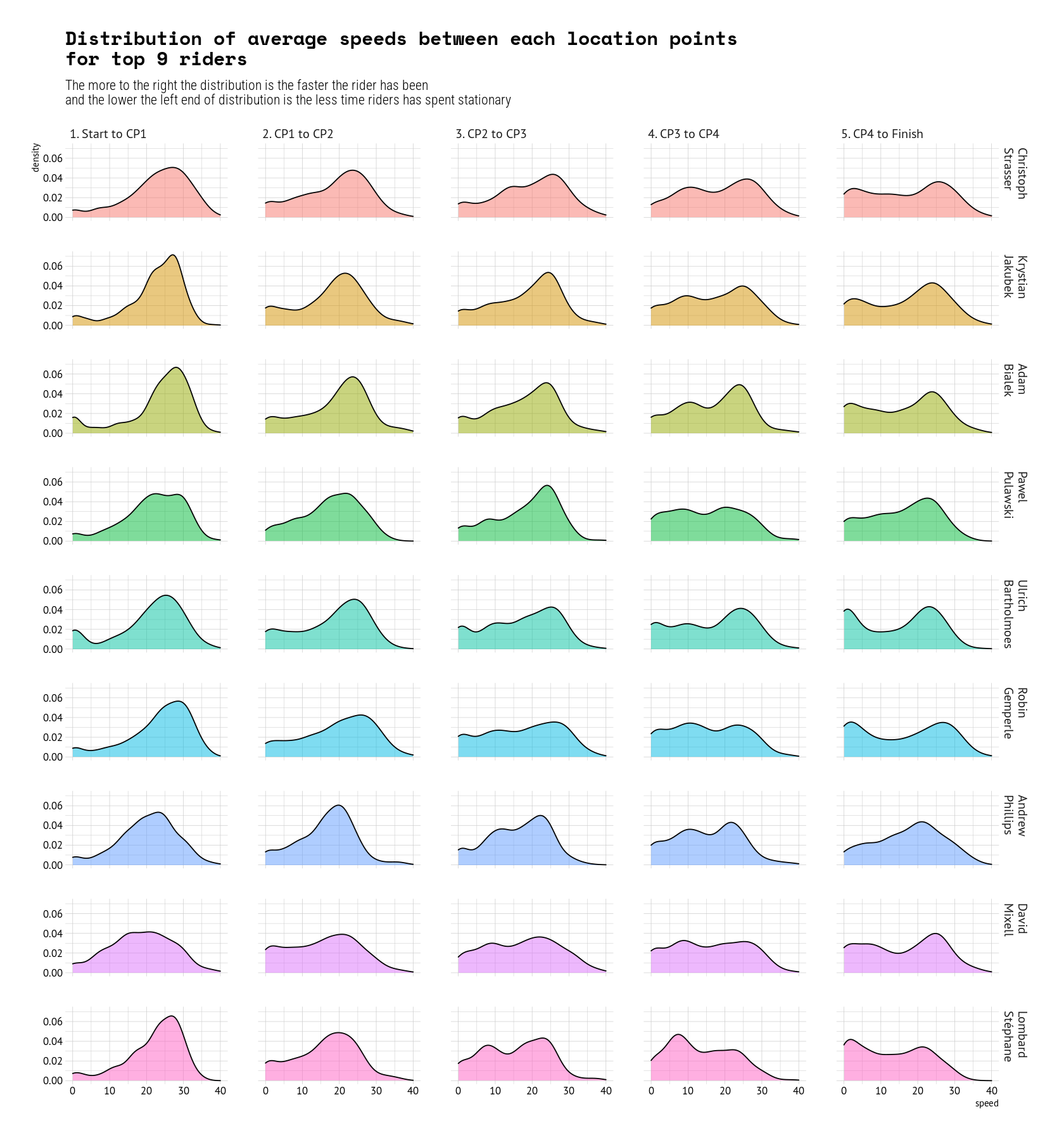# Analysing Transcontinental race 2022. Part 3: Activity patterns

data
cycling
trc
trcno8
web scraping
Tekijä

Markus Kainu

Julkaistu

5. syyskuuta 2022

In the Part 1 I demonstrated how to obtain the data from the tracking service. I Part 2 I looked at the route choices between each control points, and in this part I will look into the activity patterns of riders.

Like in the previous analysis, the key point here is to demonstrate techniques for analyzing data that tracking services provide, although I try also to answer few questions that I am interested myself when following such event.

For the analysis of activity patterns we look into each segments between location points. This means that each rider has roughly 2500 of these segments between each points. We compute distance, time and average speed for each segment and through the average speed we can indirectly reason whether rider has been moving or resting in between. Data consist only location points while rider has been moving (perhaps device only logs while moving), but in this analysis a segment with average speed less than 2km/h is considered resting and is show as blue in the graph below.

Well, what can we say. The top riders slept very little.

Koodi
``````library(dplyr)
library(sf)
library(ggplot2)
library(tidyr)
library(reactable)
library(lubridate)

riders <- unique(dat_all_points_leg\$riderName)
lst <- list()
for (i in seq(riders)){
dat_all_points_tmp <- dat_all_points_leg %>%
filter(riderName == riders[i],
scratched == 0)
lst[[i]] <- dat_all_points_tmp %>%
mutate(point_dist = c(NA,
st_distance(.[-1,],dat_all_points_tmp[-nrow(dat_all_points_tmp),],by_element=TRUE)),
point_time = difftime(time, lag(time)),
speed = point_dist/as.integer(point_time)*3.6) %>%
st_drop_geometry()
}
dat_all_points_rest <- do.call("bind_rows", lst) %>%
filter(speed < 60) %>%
mutate(mode = ifelse(speed < 2, "resting", "moving"),
)

dat_all_points_rest %>%
filter(data_quality == "good") %>%
# filter(teamNumber %in% c(100,233,75,160,50,197,01,70,10)) %>%
group_by(riderName) %>%
mutate(start = time,
day = lubridate::day(time),
finishTime2 = difftime(max(time), min(time))) %>%
ungroup() %>%
select(leg,finishTime2,riderName,mode,start,end,day) -> dat_rest

placement <- dat_rest %>%
distinct(riderName,finishTime2) %>%
arrange(finishTime2) %>%
mutate(position_overall = 1:n()) %>%
select(riderName,position_overall)

dat_activity <- left_join(dat_rest,placement)

ggplot(dat_activity %>% filter(position_overall %in% 1:9),
aes(x = start,
y = reorder(riderName, -finishTime2),
xend = end,
yend = reorder(riderName, -finishTime2),
color = mode, label = mode)) +
geom_segment(size = 5, alpha = .8) +
facet_wrap(~leg, scales = "free_x", ncol = 1) +
scale_color_manual(values = c("#b2df8a", "#1f78b4")) +
theme(legend.position = "top") +
labs(title = "Activity patterns of top 9 riders by leg")``````## Riding profiles

The term riding profile is used here to refer to distribution of average speeds in segments as described below. I use density plots instead of histograms to plot the distributions. (A density plot is a representation of the distribution of a numeric variable. It uses a kernel density estimate to show the probability density function of the variable more). Density plot is good way to present overlapping distributions of subgroups ie. riders in this case.

Just to make things clear, below is a comparison of histogram and density plot.

Koodi
``````histo_dat <- left_join(dat_all_points_rest, placement) %>%
mutate(riderName = stringr::str_wrap(riderName, width = 8),
# leg = stringr::str_wrap(leg, width = 8),
riderName = forcats::fct_reorder(riderName, position_overall))

p1 <- ggplot(histo_dat, aes(x = speed)) + geom_density() + labs(title = "Density plot")
p2 <- ggplot(histo_dat, aes(x = speed)) + geom_histogram() + labs(title = "Histogram")
patchwork::wrap_plots(list(p2,p1))``````In the plot below we have again top 9 riders and their “riding profile” of each leg. There are differences, the greatest being in the last leg when some riders had to stop and wait for the ferry.

Koodi
``````ggplot(histo_dat %>%
filter(!is.na(leg)) %>% filter(position_overall %in% 1:9),
aes(x = speed, fill = riderName)) +
# geom_histogram(alpha = .5, position = position_dodge(width = 1)) +
geom_density(alpha = .5) +
labs(title = "Distribution of average speeds between each location points \nfor top 9 riders",
subtitle = "The more to the right the distribution is the faster the rider has been
and the lower the left end of distribution is the less time riders has spent stationary") +
facet_grid(riderName~leg) +
xlim(c(0,40)) +
theme(legend.position = "none")``````When comparing the top 3 riders, we can see that Strasser has been resting perhaps slightly less and riding tiny bit more at the top speed (>35kmh) zone.

Koodi
``````ggplot(histo_dat %>%
filter(!is.na(leg)) %>% filter(position_overall %in% 1:3),
aes(x = speed, fill = riderName)) +
geom_density(alpha = .5) +
labs(title = "Distribution of average speeds \nbetween each location \npoints for top 3 riders",
subtitle = "The more to the right the distribution is the faster the rider has been
and the lower the left end of distribution is the
less time riders has spent stationary") +
facet_wrap(~leg, ncol = 1) +
xlim(c(0,40)) +
theme(legend.position = "right")``````This was all for now, I may write one more in Finnish and focus on fellow countrymen.

CC BY 4.0

## Viittaus

BibTeX-viittaus:
``````@online{kainu2022,
author = {Kainu, Markus and Kainu, Markus},
title = {Analysing Transcontinental race 2022. Part 3: Activity
patterns},
date = {2022-09-05},
url = {https://markuskainu.fi/posts/2022-09-05-tcrno8-resting},
langid = {fi}
}
``````
Viitatkaa tähän teokseen seuraavasti:
Kainu, Markus, and Markus Kainu. 2022. “Analysing Transcontinental race 2022. Part 3: Activity patterns.” September 5, 2022. https://markuskainu.fi/posts/2022-09-05-tcrno8-resting.